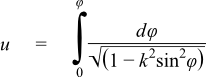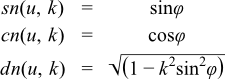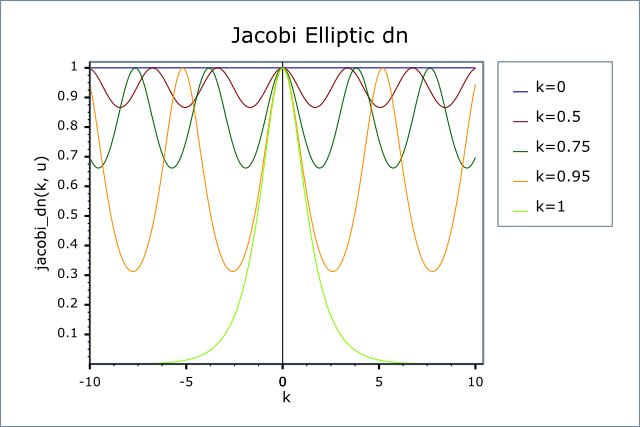#Boost C++ Libraries

...one of the most highly regarded and expertly designed C++ library projects in the world.

This is the documentation for an old version of Boost. Click here to view this page for the latest version.

### Jacobi Elliptic SN, CN and DN

##### Synopsis
```#include <boost/math/special_functions/jacobi_elliptic.hpp>
```
```namespace boost { namespace math {

template <class T, class U, class V>
calculated-result-type jacobi_elliptic(T k, U u, V* pcn, V* pdn);

template <class T, class U, class V, class Policy>
calculated-result-type jacobi_elliptic(T k, U u, V* pcn, V* pdn, const Policy&);

}} // namespaces
```
##### Description

The function jacobi_elliptic calculates the three copolar Jacobi elliptic functions sn(u, k), cn(u, k) and dn(u, k). The returned value is sn(u, k), and if provided, *pcn is set to cn(u, k), and *pdn is set to dn(u, k).

The functions are defined as follows, given:The the angle φ is called the amplitude and:Noteφ is called the amplitude. k is called the modulus.
CautionRather like other elliptic functions, the Jacobi functions are expressed in a variety of different ways. In particular, the parameter k (the modulus) may also be expressed using a modular angle α, or a parameter m. These are related by: k = sinα m = k2 = sin2α So that the function sn (for example) may be expressed as either: sn(u, k) sn(u \ α) sn(u| m) To further complicate matters, some texts refer to the complement of the parameter m, or 1 - m, where: 1 - m = 1 - k2 = cos2α This implementation uses k throughout, and makes this the first argument to the functions: this is for alignment with the elliptic integrals which match the requirements of the Technical Report on C++ Library Extensions. However, you should be extra careful when using these functions!

The final Policy argument is optional and can be used to control the behaviour of the function: how it handles errors, what level of precision to use etc. Refer to the policy documentation for more details.

The following graphs illustrate how these functions change as k changes: for small k these are sine waves, while as k tends to 1 they become hyperbolic functions:##### Accuracy

These functions are computed using only basic arithmetic operations and trigomometric functions, so there isn't much variation in accuracy over differing platforms. Typically errors are trivially small for small angles, and as is typical for cyclic functions, grow as the angle increases. Note that only results for the widest floating point type on the system are given as narrower types have effectively zero error. All values are relative errors in units of epsilon.

Table 3.29. Errors Rates in the Jacobi Elliptic Functions

Significand Size

Platform and Compiler

u < 1

Large u

53

Win32 / Visual C++ 8.0

Peak=2 Mean=0.5

Peak=44000 Mean=2500

64

Ubuntu Linux / G++ 4.7

Peak=2.0 Mean=0.5

Peak=25000 Mean=1500

##### Testing

The tests use a mixture of spot test values calculated using the online calculator at functions.wolfram.com, and random test data generated using MPFR at 1000-bit precision and this implementation.

##### Implementation

For k > 1 we apply the relations:Then filter off the special cases:

sn(0, k) = 0 and cn(0, k) = dn(0, k) = 1.

sn(u, 0) = sin(u), cn(u, 0) = cos(u) and dn(u, 0) = 1.

sn(u, 1) = tanh(u), cn(u, 1) = dn(u, 1) = 1 / cosh(u).

And for k4 < ε we have:Otherwise the values are calculated using the method of arithmetic geometric means.

 Copyright © 2006-2010, 2012, 2013 Paul A. Bristow, Christopher Kormanyos, Hubert Holin, Bruno Lalande, John Maddock, Johan Råde, Gautam Sewani, Benjamin Sobotta, Thijs van den Berg, Daryle Walker and Xiaogang Zhang Distributed under the Boost Software License, Version 1.0. (See accompanying file LICENSE_1_0.txt or copy at http://www.boost.org/LICENSE_1_0.txt)